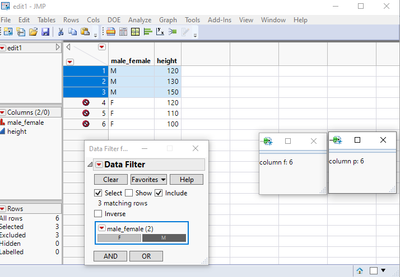Choose Language Hide Translation Bar
Highlighted

## retrieve number of rows for data table and a subset

Hi, I need to know the amount of rows that are present in the parent data table as well as the amount of rows in a filtered subset of this parent table. But when I try to retrieve both in 1 script, it only gives me the amount of rows in the parent... Can you please help?

``````//parent data table
dt = Open("C:\JMP\yield_analysis_script\used_data\edit1.jmp","visible");

//save value
f=Col Number(:height);

//print f
title1 = "parent";
text1 = Text Box ("column f: "||Char(f));
New Window(title1,text1);

//subset of parent
df = dt << Data Filter(

Mode( Include( 1 ) ),
Add Filter( columns(:male_female),Where( :male_female == "M" )));

dftest = df << subset( selected rows(1), selected columns(0));

//save value
p = Col Number(:height);

//print p
title2 = "subset";
text2 = Text Box ("column p: "||Char(p));
New Window(title2,text2);``````

This is the result I get:f and p are both equal to 6, while I expect p to be 3 (since I filtered out half of the rows..)

Does anyone see the issue here?

Thanks,

Philippe

1 ACCEPTED SOLUTION

Accepted Solutions
Highlighted

## Re: retrieve number of rows for data table and a subset

You are not pointing to your primary data table when you are doing your subset.  You are specifying

``dftest = df << subset( selected rows(1), selected columns(0));``

"df" is the pointer to the data filter not the data table.  You should be specifying

``dftest = dt << subset( selected rows(1), selected columns(0));``

Also, a better way to find the number of rows in a data table is to use the N Rows() function, not the Col Number().

``````numRowsMain = N Rows( dt );
// and/or
numRowsSub = N Rows( dftest );``````
Jim
Highlighted

## Re: retrieve number of rows for data table and a subset

You are not pointing to your primary data table when you are doing your subset.  You are specifying

``dftest = df << subset( selected rows(1), selected columns(0));``

"df" is the pointer to the data filter not the data table.  You should be specifying

``dftest = dt << subset( selected rows(1), selected columns(0));``

Also, a better way to find the number of rows in a data table is to use the N Rows() function, not the Col Number().

``````numRowsMain = N Rows( dt );
// and/or
numRowsSub = N Rows( dftest );``````
Jim
Article Labels